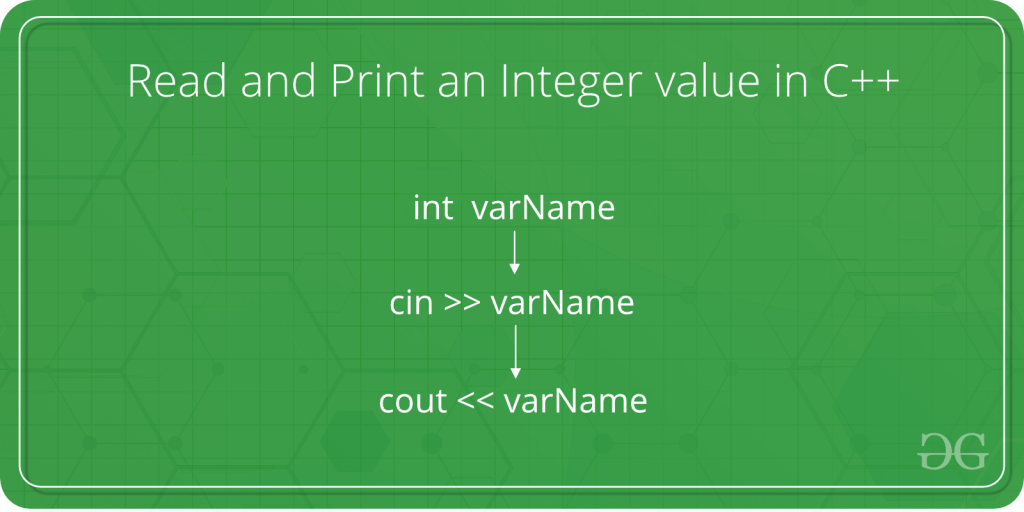# How to Read and Print an Integer value in C++

• Last Updated : 05 Dec, 2018

The given task is to take an integer as input from the user and print print that integer in C++ language.Attention reader! All those who say programming isn't for kids, just haven't met the right mentors yet. Join the  Demo Class for First Step to Coding Coursespecifically designed for students of class 8 to 12.

The students will get to learn more about the world of programming in these free classes which will definitely help them in making a wise career choice in the future.

In below program, the syntax and procedures to take the integer as input from the user is shown in C++ language.

Steps:

1. The user enters an integer value when asked.
2. This value is taken from the user with the help of cin method. The cin method, in C++, reads the value from the console into the specified variable.

Syntax:

```cin >> variableOfXType;

where >> is the extraction operator
and is used along with the object cin
for reading inputs. The extraction operator
extracts the data from the object cin
which is entered using the keyboard.
```
3. For an integer value, the X is replaced with type int. The syntax of cin method becomes as follows then:

Syntax:

```cin >> variableOfIntType;
```
4. This entered value is now stored in the variableOfIntType.
5. Now to print this value, cout method is used. The cout method, in C++, prints the value passed as the parameter to it, on the console screen.

Syntax:

```cout << variableOfXType;

where << is the insertion operator.
The data needed to be displayed on the screen
is inserted in the standard output stream (cout)
using the insertion operator (<<).
```
6. For an integer value, the X is replaced with type int. The syntax of cout() method becomes as follows then:

Syntax:

```cout << variableOfIntType;
```
7. Hence, the integer value is successfully read and printed.

Program:

## C++

 `// C++ program to take an integer``// as input and print it`` ` `#include ``using` `namespace` `std;`` ` `int` `main()``{`` ` `    ``// Declare the variables``    ``int` `num;`` ` `    ``// Input the integer``    ``cout << ``"Enter the integer: "``;``    ``cin >> num;`` ` `    ``// Display the integer``    ``cout << ``"Entered integer is: "` `<< num;`` ` `    ``return` `0;``}`

Output:

```Enter the integer: 10
Entered integer is: 10
```
My Personal Notes arrow_drop_up